# Average Function in Microsoft Excel

In Excel, AVERAGE function is one of the statistical and most commonly used functions. It is the average (arithmetic mean) of the numbers provided in the argument. AVERAGE is calculated by adding the sum of the cells and then dividing the total by the number of cells in the argument.

With the help of examples let us understand the use of Average function in Excel.

Refer to these 7 different examples for the usage of Average function in Microsoft Excel on the basis of following sample data: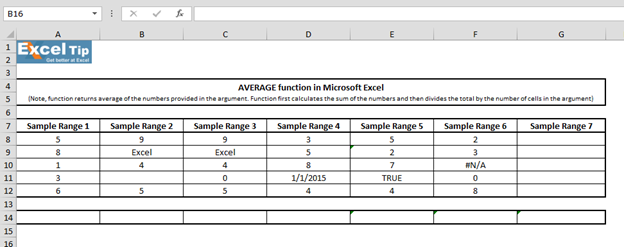1st Example:

In the sample 1 we have few numbers for which we want to calculate average. Follow the steps given below:

• Enter AVERAGE function in cell A14
• =AVERAGE(A8:A12,7)
• Press Enter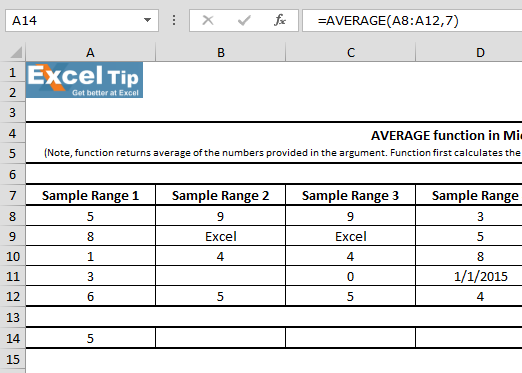Note: It can take up to 255 number of arguments in Excel 2007 or later versions.

Formula Explanation: A8 to A12 as range in the first argument, then we close the parentheses and hit enter. The function returns 4.6 as average of these 5 numbers. Also, if you sum up these numbers 5 + 8 + 1 + 3 + 6 it will be equal to 23. And, as we have 5 numbers of cells in the argument, and when we divide 23 by 5, it returned 4.6 which as an average.

2nd Example:

In this example, we’ll see how AVERAGE function handles any empty cell or cells that contain text in the range, follow the steps given below:

• Enter AVERAGE function in cell B14
• Then, select B8:B12 as range
• =AVERAGE(B8:B12)
• Press Enter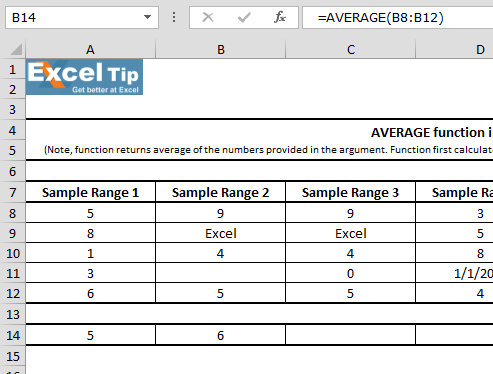Formula Explanation: In this case function returns 6 as average. Because cell B9 is ignored by AVERAGE function, since it contains text instead of a number and function found no value in B11.

3rd Example:

In this example, we’ll learn if there is a cell that contains zero in the range. Follow the steps given below:

• Enter function in cell C14
• Select C8 to C12 range
• =AVERAGE(C8:C12)
• Press Enter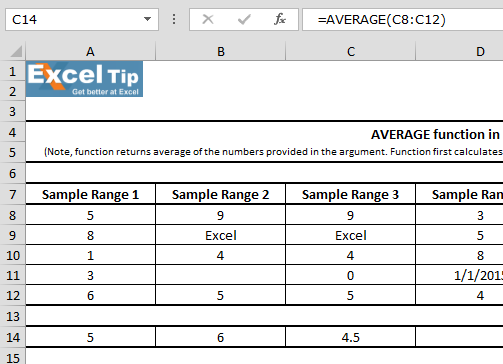4th Example:

In this example, we can see that one of the cell contains date within a range. So, we’ll learn that how Average function will work in case of any cell that contains date.

• Enter the function in cell D14
• =AVERAGE(D8:D12)
• Press Enter
• Function returns 8405. It is because in Excel, dates are saved as numbers. In this example, we have taken 1st Jan,2015 which is equivalent to 420055th Example:

In this example, we have taken text in the range for which we want to calculate average.

• Enter the function in cell E14
• =AVERAGE(E8:E12)
• Press Enter
• Function returns 4.5 as result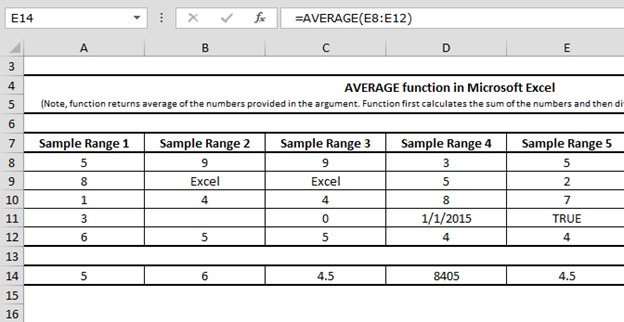It means Average function does not consider text value while performing the calculation. And by doing so it provides accurate result.

6th Example:

In this example, we’ll learn if a cell is having #N/A error within a range for which we want to calculate average, then how Average function will perform.

• Enter the function in cell F14
• =AVERAGE(F8:F12)
• Press Enter
• Function will return #N/Aas result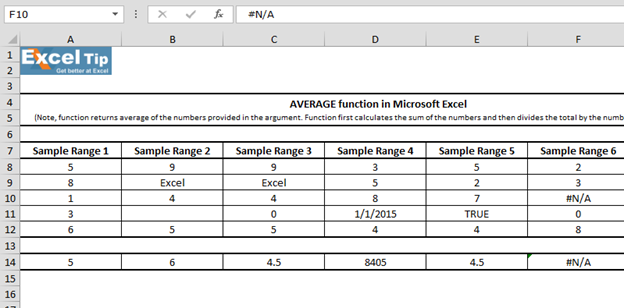If any cell contains an error, the formula would also return error.

7th Example:

In this example, we’ll check if selected range for average function is blank and how Average function will work.

• Enter the function in cell G14
• =AVERAGE(G8:G12)
• Press Enter

The function will return #DIV/0! Error because function did not find any value to be averaged in the argument.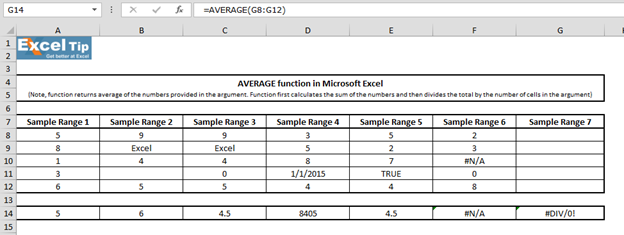In this way we can make use of average function in MS-Excel.# Video: How to use AVERAGE function in Excel

Watch the steps in this short video, and the written instructions are above the video

We would love to hear from you, do let us know how we can improve, complement or innovate our work and make it better for you. Write us at info@exceltip.com

## Users are saying about us...

1.Hi! can you explain your requirement in detail.

Terms and Conditions of use

The applications/code on this site are distributed as is and without warranties or liability. In no event shall the owner of the copyrights, or the authors of the applications/code be liable for any loss of profit, any problems or any damage resulting from the use or evaluation of the applications/code.